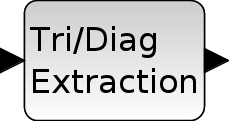Change language to:
English - Français - 日本語 - Русский

See the recommended documentation of this function

Ajuda do Scilab >> Xcos > palettes > Matrix_pal > EXTTRI

# EXTTRI

Triangular or Diagonal Extraction

### Block Screenshot### Description

The EXTTRI block extracts some elements from the input matrix u. The size of the output matrix is the same that those of the input matrix.

When the extraction type parameter is set to:

• 1 (Upper triangle): the block copies the elements on and above the main diagonal to an output matrix. The elements below the main diagonal are set to zero.

• 2 (Lower triangle): the block copies the elements on and below the main diagonal to an output matrix. The elements above the main diagonal are set to zero.

• 3 (Diagonal): the block copies the elements on the main diagonal to an output matrix. The elements above and below the main diagonal are set to zero.

Equivalent functions of EXTTRI in Scilab are triu(u), tril(u) and diag(u), respectively

### Parameters• Datatype(1=real double 2=Complex)

It indicates the type of the output. It support only the two types double (1) and complex (2). If we input another entry in this label xcos will print the message "Datatype is not supported".

Properties : Type 'vec' of size 1.

• Extraction type (1=lower 2=upper 3=diagonal)

It indicates the form of the output matrix. It can be an upper triangle, a lower triangle or a diagonal matrix.

Properties : Type 'vec' of size 1.

### Default properties

• always active: no

• direct-feedthrough: yes

• zero-crossing: no

• mode: no

• regular inputs:

- port 1 : size [-1,-2] / type 1

• regular outputs:

- port 1 : size [-1,-2] / type 1

• number/sizes of activation inputs: 0

• number/sizes of activation outputs: 0

• continuous-time state: no

• discrete-time state: no

• object discrete-time state: no

• name of computational function: extrilz

### Example

```A = [1 2 3 ; 4 5 6 ; 7 8 9 ; 10 11 12]
If the extraction type is 2 then the output is
B = [1 0 0 ; 4 5 0 ; 7 8 9 ; 10 11 12]
```

Example of diagonal extraction### Interfacing function

• SCI/modules/scicos_blocks/macros/MatrixOp/EXTTRI.sci

### Computational function

• SCI/modules/scicos_blocks/src/c/exttril.c
• SCI/modules/scicos_blocks/src/c/exttriu.c
• SCI/modules/scicos_blocks/src/c/extdiag.c
• SCI/modules/scicos_blocks/src/c/exttrilz.c
• SCI/modules/scicos_blocks/src/c/exttriuz.c
• SCI/modules/scicos_blocks/src/c/extdiagz.c